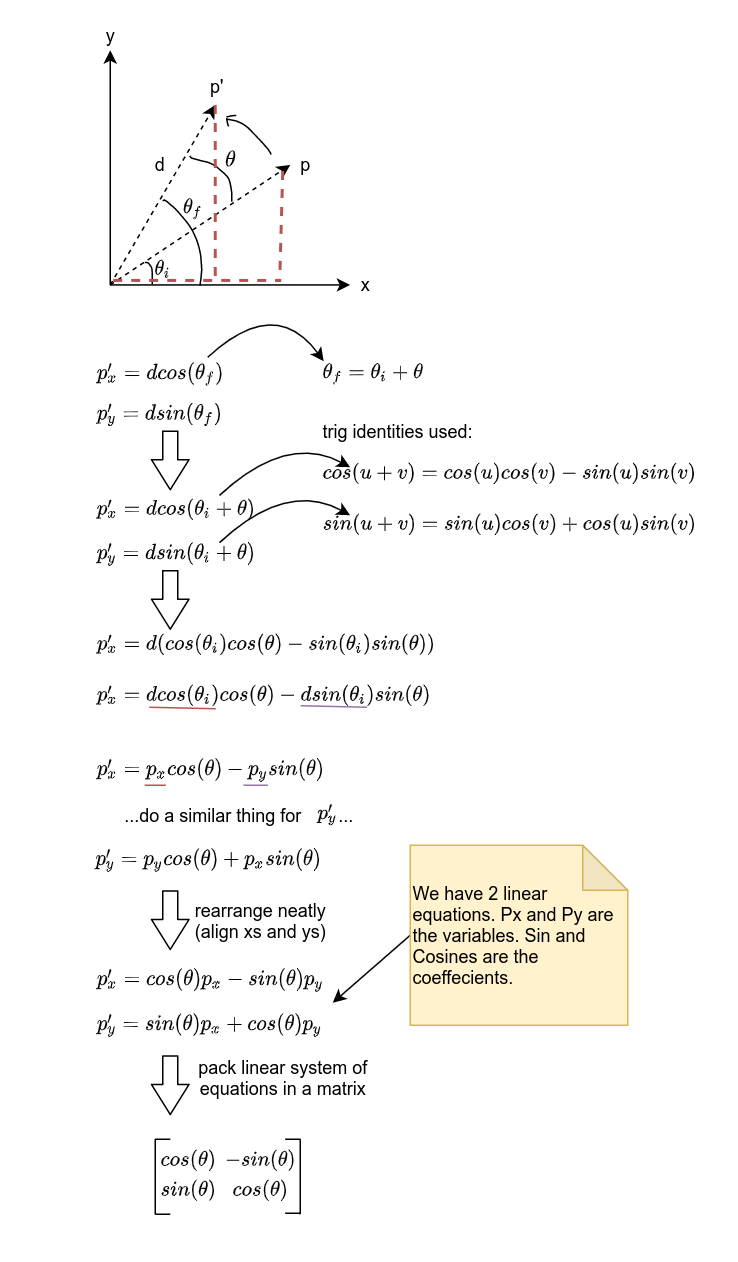You have some point $p$ and you want it to rotate $\theta$ degrees about the origin (0,0). We already did this here, but let’s try a different way, one that we can pack in a matrix (thus our equations need to be linear).

Once again, we draw the situation (including the knowns, unknowns, and any needed auxillary elements). When we get to the point where we are adding angles inside sins and cosines, we will use two trig identities to “simplify” the expression (simplify in the sense that our equations become linear).Here is an implementation of the above solution in iodide.